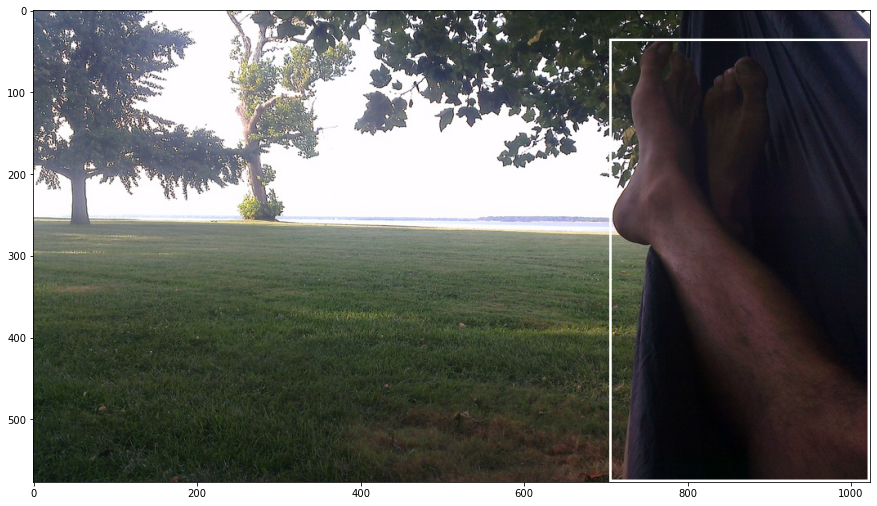#### AI Blitz 5 ⚡

Augmentation to improve baseline score

We have used Detectron2, Facebook AI Research’s next-generation software system that implements state-of-the-art object detection algorithms.

# OBJDE submission¶

Team: HARD_DRIVE_CORRUPTED

### Install Pre-Built Detectron2¶

In [ ]:
!python3 -m pip install detectron2 -f https://dl.fbaipublicfiles.com/detectron2/wheels/cu102/torch1.7/index.html


### Import Libraries¶

In [ ]:
# Some basic setup:
# Setup detectron2 logger
import torch, torchvision

import detectron2
from detectron2.utils.logger import setup_logger
setup_logger()

# import some common libraries
import numpy as np
import os, json, cv2, random

# import some common detectron2 utilities
from detectron2 import model_zoo
from detectron2.engine import DefaultPredictor
from detectron2.config import get_cfg
from detectron2.utils.visualizer import Visualizer
from detectron2.structures import BoxMode

import pandas as pd
import numpy as np
from PIL import Image, ImageDraw
import matplotlib.pyplot as plt
from tqdm.notebook import tqdm
import matplotlib.pyplot as plt
import itertools


### Dataset¶

The dataset consists of images of the 5 classes Person, Clothing, Car, Plant and Footwear. There are 40000 training and 4000 validation images. The CSV files containes the labels and bounding box(XMin, XMax, YMin and YMax) of images normalised between 0 and 1. There can be multiple objects in an image.

In [ ]:
train_df = pd.read_csv("../input/object-det/train.csv")

In [ ]:
# labels to number mapping
category_dict = {"Person":0, "Clothing":1, "Car":2, "Plant":3, "Footwear":4}

In [ ]:
train_df['category_id'] = train_df['LabelName'].apply(lambda x : category_dict[x])
train_df['image_category_id'] = train_df.groupby(['ImageID']).ngroup()

val_df['category_id'] = val_df['LabelName'].apply(lambda x : category_dict[x])
val_df['image_category_id'] = val_df.groupby(['ImageID']).ngroup()


### Data visualisation¶

In [ ]:
def show_images(images, num = 5):

images_to_show = np.random.choice(images, num)

for image_id in images_to_show:

image = Image.open(f'../input/object-det/train_repaired/train/{image_id}.jpg')

w, h = image.size

#         print(w, h)

bboxes = train_df[train_df['ImageID'] == image_id][['XMin','XMax','YMin','YMax']].to_numpy()

# visualize them
draw = ImageDraw.Draw(image)
for bbox in bboxes:
draw.rectangle([bbox*w, bbox*h, bbox*w, bbox*h], width=3)

plt.figure(figsize = (15,15))
plt.imshow(image)
plt.show()

show_images(train_df['ImageID'].unique(), num = 3)